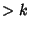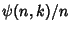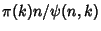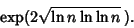## Smooth Number

An Integer is-smooth if it has no Prime Factors. The probability that a random Positive Integeris-smooth is, whereis the number of-smooth numbers. This fact is important in application of Kraitchik's extension of Fermat's Factorization Method because it is related to the number of random numbers which must be examined to find a suitable subset whose product is a square.

Since about-smooth numbers must be found (whereis the Prime Counting Function), the number of random numbers which must be examined is about. But because it takes aboutsteps to determine if a number is-smooth using Trial Division, the expected number of steps needed to find a subset of numbers whose product is a square is(Pomerance 1996). Canfield et al. (1983) showed that this function is minimized whenand that the minimum value is aboutIn the Continued Fraction Factorization Algorithm,can be taken as, but in Fermat's Factorization Method, it is.is an estimate for the largest Prime in the Factor Base (Pomerance 1996).

References

Canfield, E. R.; Erdös, P.; and Pomerance, C. On a Problem of Oppenheim Concerning Factorisation Numerorum.''' J. Number Th. 17, 1-28, 1983.

Pomerance, C. On the Role of Smooth Numbers in Number Theoretic Algorithms.'' In Proc. Internat. Congr. Math., Zürich, Switzerland, 1994, Vol. 1 (Ed. S. D. Chatterji). Basel: Birkhäuser, pp. 411-422, 1995.

Pomerance, C. `A Tale of Two Sieves.'' Not. Amer. Math. Soc. 43, 1473-1485, 1996.